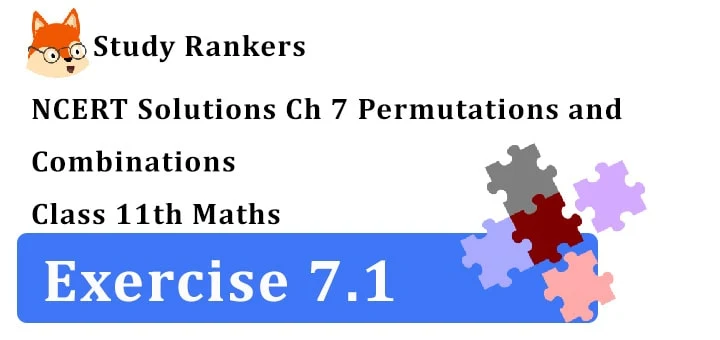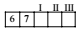## NCERT Solutions for Class 11 Maths Chapter 7 Permutations and Combinations Exercise 7.1

Chapter 7 Permutations and Combinations Exercise 7.1 Class 11 Maths NCERT Solutions are very helpful in practicing math questions and getting significant marks in the examinations. NCERT Solutions for Class 11 Maths will be useful for you if you want to complete your homework or know the application of formulas. This will guide prove useful if you want to score good marks in the exams.1. How many 3-digit numbers can be formed from the digits 1, 2, 3, 4 and 5 assume that
(i) repetition of the digits is allowed
(ii) repetition of the digits is not allowed.

(i) There are five digits viz 1, 2, 3, 4 and 5. Every digit can be selected any number of times. Hence, we can select first digit in 5 ways. The second digit in 5 ways and the third digit in 5 ways. Hence, the number of ways in which the selection of three digits can be made = 5 × 5 × 5 ways = 125 ways.

(ii) Under the restriction, first digit can be selected 5 ways. After the selection of first digit four digits are left. Second digit can be selected in 4 ways and third digit can be selected in 3 ways. The total number of ways of selecting three digits is 5 × 4 × 3 = 60.

2. How many 3-digit even numbers can be formed from the digits 1, 2, 3, 4, 5, 6 if the digits can be repeated?

Let 2 be fixed at unit’s place. The ten’s place can be filled up in 6 ways. The hundred’s place can be filled in 6 ways. Number of numbers that can be formed when 2 is at units place = 6 × 6 =36. Similarly when 4 is at unit’s place, the number of numbers that can be formed = 36. Again when 6 is at the unit’s place. The number of numbers that can be formed = 36.
∴ the total number of ways when 3 digit numbers can be formed, the digits being repeated = 36 × 3 = 108

3. How many 4-letter code words are possible using the first 10 letter of the English alphabet if no letter can be repeated?

First letter of the code word can be selected in 10 ways. After the selection of the first letter, we have 9 letters. Hence, the second letter can be selected in 9 ways. According to fundamental principle of counting (FPC), first two letters can be selected in 10 × 9 ways. Similarly, third letter can be selected in 8 ways and the fourth letter can be selected in 7 ways. According to FPC, number of ways of selecting four letters out of 10 letters of English alphabet
= 10 × 9 × 8 × 7 ways = 5040 ways.

4. How many 5-digit telephone numbers can be constructed using the digits 0 to 9 if each number starts with 67 and no digit appears more than once.

There are 10 digits from 0 to 9I. place after 67 can be filled in 8 ways
II. place after 67 can be filled in 7 ways
III. place after 67 can be filled in 6 ways
∴ No. of telephone numbers that can be constructed
= 8 × 7 × 6 = 336

5. A coin is tossed 3 times and the outcomes are recorded. How many possible outcomes are there?

In tossing a coin there are two possible outcomes. via. H or T (i.e., Head and tail) Similarly, tossing the coin for the second time, there are again the same two outcome and similarly for third toss the same outcomes appear.
Total number of outcomes in three toss
= 2 × 2 × 2 = 8

6. Given 5 flags of different colours, how many different signals can be generated if each signal requires the use of 2 flags, one below the other?

First place can be filled up in 5 ways.
After filling first place we are left with 4 place
the second place can be filled up in 4 ways .
The total number of signals which can be generated = 5 × 4 = 20
=>  8/7 = x/8.7 => x = 64

7. Evaluate n! /(n –r) - when
(i) n = 6, r = 2
(ii) n = 9, r = 5